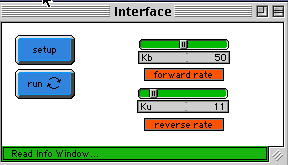WHAT IS IT?
-----------

This model demonstrates the kinetics of a reversible reaction of the type:

Kb
A + A <=======> B
Ku

An example of such a reaction would be dimerization of acetic acid:

Kb
2 H C-COOH <=======> H C-COOH~...~HOOC-C H
3           Ku     3                 3

This reaction is an example of a complex reaction which consists of two
elementary reactions.  The forward bimolecular reaction

Kb
A + A --------> B

is characterized by the constant Kb and the reverse unimolecular reaction

Ku
B ---------> A + A

is chracterized by the constant Ku.

The system of ordinary differential equations (ODE) that describes the
concentrations of A and B is given below:

dA           2
-- = -2Kb * A  + 2Ku * B		(1)
dt

dB         2
-- = Kb * A  - Ku * B		(2)
dt

The usual initial conditions are A(0) = Ao and B(0) = 0.  While it is
possible to solve this system of ODE analytically, chemists usually
apply the Principle of Stationary Concentrations when they investigate
the kinetics of reactions of this type.  The Principle says that one can
assume that the concentrations of the species stop changing from some point
on after the system reaches equilibrium.  If concentrations are stationary,
the derivatives

dA	 dB
--  and	 --
dt	 dt

are zero.  Hence one can replace the system of ODE above with the system
of algebraic equations below:
2
0 = -2Kb * A*   + 2Ku * B* 		(1')

2
0 = Kb * A*  - Ku * B*  		(2')

where concentrations marked with * are stationary concentrations.  The
second equation (2') is linearly dependent on the first equation (1').
Luckily we also have another equation coming from the law of the conservation
of mass:

A* + 2 * B* = Ao			(3)

From equation (2') we can express B* in terms of A* :

Kb     2
B*  = --  A* 			(4)
Ku

We can now plug in expression (4) into (3) and then we will have a quadratic
equation in terms of A*:

Kb   2
A*  + -- A*  = Ao 		(5)
Ku

whose solution is:

_____________
|
|	        Kb
|  1 + 4 * --   - 1
\|          Ku
--------------------		(6)
Kb
2 * --
Ku

One can now find the stationary concentration of B using equation (4).

This model demonstrates numerically that the appliction of the Principle
of Stationary Concentrations is valid in this case.  In this model there
are two breeds (green turtles and red turtles)  Green turtles turn into red
turtles bimolecularly whereas red turtles turn back into pairs of green turtles
monomolecularly.  The user can control the rate at which this changes occur with
sliders kb and Ku.   if you ran the program long enough with both Ku and Kb values
being non-zero you will observe that the plots of concentrations become stationary.

HOW TO USE IT
--------------

Choose the values of Ku and Kb with appropriate sliders:
- Kb controls the rate of the forward reaction by which two green turtles
turn bimolecularly into a single red turtle.
- Ku controls the rate of the reverse reaction, by which a red turtle
turns unimolecularly into two green turtles.

Having chosen appropriate values of the constants, press SETUP to clear
the screen and create an initial number of green turtles.  Note: we do
not create red turtles initially, although this can be done in principle.

Press RUN to start the simulation.

THINGS TO NOTICE
----------------

You will see turtles wandering around the screen and  changing color.  Pay
more attention to the plot of the concentrations.  Do the plots soon reach
stationary concentrations?

THINGS TO TRY
-------------

How do the stationary concentrations depend on the values of Kb and Ku?
You can change Ku and Kb while the model is running.   See if you can
predict what the stationary concentrations will be with various combinations
of Kb and Ku.

EXTENDING THE MODEL
------------------

Try to implement the following reaction:

Kb         K2
A + A <======> B -------> C
Ku

This reaction underlines a vast number of microbiological processes
(e.g. fermentation).  You can read about its kinetics in any book on
Biochemistry.  Look up the so-called Michaeles-Menten equation.  Does
it check numerically?

Try to implement the following reaction:

Kb         K2
A + B <======> C -------> D
Ku

STARLOGOT FEATURES
------------------

The major subroutines are listed below.  Notice how much more complicated
the implementation of "to react-forward" is.  This is hardly suprising
considering that it is in general harder to design models where agents
interact with each other as opposed to models where agents act independently.

to react-forward
if (count-init-breed-here > 1) and ((random 999) > 998 - Kb) and (id = nobody)
[
setid one-of-turtles-here
ifelse (id-of id) != nobody [setid nobody][setid-of id who]]

if id != nobody [
setbreed prod-breed
setcolor red
if who < id [setvalue value + value-of id setvalue-of id value]]
end

to react-backward
if (random 999) > (999 - Ku) [
setbreed init-breed
setcolor green

; This line seems to be unneccessary but without it the code does not work right.

setid nobody
hatch [setvalue 1 setid nobody]
hatch [setvalue 1 setid nobody]
die]
end

A good indication that this code works correctly is the constancy of

sum-of-turtles [value]

If you look closely at the implementation you will see that green turtles
always carry value equal to one unlike red turtles which always have value two.
Even though it takes two green turtles to produce a red turtle, the sum of values
stays the same.  This corresponds to the pricnciple of conservation of mass
(equation 3).